Theory and Modern Applications

# Existence of solutions to strongly damped quasilinear wave equations

## Abstract

In this paper, we study the strongly damped quasilinear wave equation. By using spatial sequence techniques and energy estimate methods, we obtain the existence theorem of the solution to abstract a strongly damped wave equation and to a class of strongly damped quasilinear wave equations.

MSC:35L05, 35L20, 35D30, 35D35.

## 1 Introduction

This paper is concerned with the following initial-boundary problem of strongly damped quasilinear wave equations:

$\left\{\begin{array}{c}\frac{{\partial }^{2}u}{\partial {t}^{2}}-k\frac{\partial \mathrm{\Delta }u}{\partial t}=-{\sum }_{|\alpha |\le m}{\left(-1\right)}^{|\alpha |}{D}^{\alpha }{A}_{\alpha }\left(x,u,\dots ,{D}^{m}u\right)+g\left(x,u,\dots ,{D}^{m}u\right),\hfill \\ u{|}_{\partial \mathrm{\Omega }}=\cdots ={D}^{m-1}u{|}_{\partial \mathrm{\Omega }}=0,\hfill \\ u\left(x,0\right)=\phi ,\phantom{\rule{2em}{0ex}}{u}_{t}\left(x,0\right)=\psi ,\hfill \end{array}$
(1.1)

where $k>0$, $m\ge 1$, Δ is the Laplacian operator, Ω denotes an open bounded set of ${R}^{n}$ with smooth boundary Ω, and u denotes vertical displacement at $\left(x,t\right)$.

Equation (1.1) is a quasilinear wave equation with strong damping, which has many applications. The existence and asymptotic behavior for the strongly damped wave equations have been extensively studied by many authors . Local well-posedness for strongly damped wave equations with critical nonlinearities is studied in . The existence and asymptotic behavior for a strongly damped nonlinear wave equation have been concerned in [1, 39, 1215]. Fan  investigated the existence and the continuity of the inflated attractors for a class of nonautonomous strongly damped wave equations through differential inclusion. Li  obtained the existence of a global periodic attractor attracting any bounded set exponentially in the phase space by introducing a new norm, which is equivalent to the usual norm.

The quasilinear wave equation has been investigated by many authors in the last years . In , it is considered the boundary value problem for the quasilinear wave equation. Under certain assumptions, the global smooth solvability is obtained. It has been shown by Alinhac [21, 22] that the null condition implies global existence of smooth solutions in two space dimensions. Zhang  studies the global existence, singularities, and life span of smooth solutions of the Cauchy problem for a class of quasilinear hyperbolic systems with higher order dissipative terms and gives their applications to nonlinear wave equations with higher order dissipative terms. Metcalfe and Sogge  give a simple proof of global existence for quadratic quasilinear Dirichlet-wave equations outside of a wide class of compact obstacles in the critical case where the spatial dimension is three. Yin  gives the lower bound of a lifespan of classical solutions and discusses the long time asymptotic behavior of solutions away from the blowup time. Weidemaier  establishes local (in time) existence of classical solutions to the initial-boundary value problem for a quasilinear wave equation. In , the existence and uniqueness of the classical solutions for the initial value problems and the first boundary problems of a quasilinear wave equation are proved by the Galerkin method. In , the numerical solution for a type of quasilinear wave equation is studied. The three-level difference scheme for quasilinear waver equation with strong dissipative term is constructed and the convergence is proved.

The strongly damped wave equations and the quasilinear wave equation have a lot of results. But up to now, we find several results on the strongly damped quasilinear wave equation. Chen  shows that the initial boundary value problem for the strongly damped quasilinear wave equation has a global solution and that there exists a compact global attractor with finite dimension. Comparing Eq. (1.1) and , we find that ${A}_{\alpha }\left(x,u,\dots ,{D}^{m}u\right)=\sigma {\left({u}_{x}\right)}_{x}$, $g\left(x,u,\dots ,{D}^{m}u\right)=-f\left(u\right)+g\left(x\right)$, and $x\in \mathrm{\Omega }=\left[0,1\right]$. In this article, our interest is to study that Eq. (1.1) has a solution under which condition of A and g. This article uses the spatial sequence techniques, each side of the equation to be treated in different spaces, which is an important way to get more extensive and wonderful results.

The outline of the paper is as follows. In Section 2, we provide essential preliminaries, which include definitions and lemmas from . In Section 3, we give existence of solutions to abstract strongly damped wave equations. In Section 4, we present the main results and their proof. Existence of solutions to a class of strongly damped quasilinear wave equations is given.

## 2 Preliminaries

We introduce two spatial sequences:

$\left\{\begin{array}{c}X\subset {H}_{3}\subset {X}_{2}\subset {X}_{1}\subset H,\hfill \\ {X}_{2}\subset {H}_{2}\subset {H}_{1}\subset H,\hfill \end{array}$
(2.1)

where H, ${H}_{1}$, ${H}_{2}$, ${H}_{3}$ are Hilbert spaces, X is a linear space, and ${X}_{1}$, ${X}_{2}$ are Banach spaces. All imbeddings of (2.1) are dense. Let

$\left\{\begin{array}{c}L:X\to {X}_{1}\phantom{\rule{1em}{0ex}}\text{is one-one dense linear operator},\hfill \\ {〈Lu,v〉}_{H}={〈u,v〉}_{{H}_{1}},\phantom{\rule{1em}{0ex}}\mathrm{\forall }u,v\in X.\hfill \end{array}$
(2.2)

Furthermore, L has eigenvectors $\left\{{e}_{k}\right\}$ satisfying

$L{e}_{k}={\lambda }_{k}{e}_{k}\phantom{\rule{1em}{0ex}}\left(k=1,2,\dots \right),$
(2.3)

and $\left\{{e}_{k}\right\}$ constitutes a common orthogonal basis of H and ${H}_{3}$.

We consider the following abstract equation:

$\left\{\begin{array}{c}\frac{{d}^{2}u}{d{t}^{2}}+k\frac{d}{dt}\mathcal{L}u=G\left(u\right),\phantom{\rule{1em}{0ex}}k>0,\hfill \\ u\left(0\right)=\phi ,\phantom{\rule{2em}{0ex}}{u}_{t}\left(0\right)=\psi ,\hfill \end{array}$
(2.4)

where $G:{X}_{2}×{R}^{+}\to {X}_{1}^{\ast }$ is a map, ${R}^{+}=\left[0,\mathrm{\infty }\right)$ and $\mathcal{L}:{X}_{2}\to {X}_{1}$ is a bounded linear operator satisfying

${〈\mathcal{L}u,Lv〉}_{H}={〈u,v〉}_{{H}_{2}},\phantom{\rule{1em}{0ex}}\mathrm{\forall }u,v\in {X}_{2}.$
(2.5)

Definition 2.1 We say $u\in {W}^{1,\mathrm{\infty }}\left(\left(0,T\right),{H}_{1}\right)\cap {L}^{\mathrm{\infty }}\left(\left(0,T\right),{X}_{2}\right)$ is a global weak solution of Eq. (2.4) provided that $\left(\phi ,\psi \right)\in {X}_{2}×{H}_{1}$

${〈{u}_{t},v〉}_{H}+k{〈\mathcal{L}u,v〉}_{H}={\int }_{0}^{t}〈G\left(u\right),v〉\phantom{\rule{0.2em}{0ex}}dt+{〈\psi ,v〉}_{H}+k{〈\mathcal{L}\phi ,v〉}_{H},$
(2.6)

for all $v\in {X}_{1}$ and $0\le t\le T<\mathrm{\infty }$.

Definition 2.2 Let ${u}_{n},{u}_{0}\in {L}^{p}\left(\left(0,T\right),{X}_{2}\right)$. We say ${u}_{n}⇀{u}_{0}$ in ${L}^{p}\left(\left(0,T\right),{X}_{2}\right)$ is uniformly weakly convergent if $\left\{{u}_{n}\right\}\subset {L}^{p}\left(\left(0,T\right),H\right)$ is bounded, and

(2.7)

Definition 2.3 We say that a map $G:{X}_{2}×\left(0,\mathrm{\infty }\right)\to {X}_{1}^{\ast }$ is T-coercive weakly continuous if for all $\left\{{u}_{n}\right\}\subset {L}_{\mathrm{loc}}^{p}\left(\left(0,\mathrm{\infty }\right),{X}_{2}\right)\cap {L}_{\mathrm{loc}}^{\mathrm{\infty }}\left(\left(0,\mathrm{\infty }\right),H\right)$, ${u}_{n}⇀{u}_{0}$ in ${L}^{p}\left(\left(0,T\right),{X}_{2}\right)$ is uniformly weakly convergent, and

$\underset{n\to \mathrm{\infty }}{lim}{\int }_{0}^{t}|〈G{u}_{n}-G{u}_{0},L{u}_{n}-L{u}_{0}〉|\phantom{\rule{0.2em}{0ex}}dt=0,\phantom{\rule{1em}{0ex}}0

then

$\underset{n\to \mathrm{\infty }}{lim}{\int }_{0}^{t}|〈G{u}_{n},v〉|\phantom{\rule{0.2em}{0ex}}dt=\underset{n\to \mathrm{\infty }}{lim}{\int }_{0}^{T}|〈G{u}_{0},v〉|\phantom{\rule{0.2em}{0ex}}dt,\phantom{\rule{1em}{0ex}}\mathrm{\forall }v\in {X}_{1},0

Lemma 2.4 ()

Let$\left\{{u}_{n}\right\}\in {L}^{p}\left(\left(0,T\right),{W}^{m,p}\left(\mathrm{\Omega }\right)\right)$ ($m\ge 1$) be bounded sequences, and$\left\{{u}_{n}\right\}$uniformly weakly convergent to$\left\{{u}_{0}\right\}\in {L}^{p}\left(\left(0,T\right),{W}^{m,p}\left(\mathrm{\Omega }\right)\right)$. Then, for all$|\alpha |\le m-1$, it follows that

${D}^{\alpha }{u}_{n}\to {D}^{\alpha }{u}_{0}\phantom{\rule{1em}{0ex}}\mathit{\text{in}}\phantom{\rule{0.25em}{0ex}}{L}^{2}\left(\left(0,T\right)×\mathrm{\Omega }\right).$
(2.8)

Lemma 2.5 ()

Let$\mathrm{\Omega }\subset {R}^{n}$be a open set, and$f:\mathrm{\Omega }×{R}^{N}\to {R}^{1}$satisfy the Caratheodory condition and

$|f\left(x,\xi \right)|\le C\sum _{i=1}^{N}{|{\xi }_{i}|}^{\frac{{p}_{i}}{p}}+b\left(x\right).$
(2.9)

If$\left\{{u}_{{i}_{k}}\right\}\subset {L}^{{p}_{i}}\left(\mathrm{\Omega }\right)$ ($1\le i\le N$) is bounded and${u}_{{i}_{k}}$convergent to${u}_{i}$in${\mathrm{\Omega }}_{0}$for all bounded${\mathrm{\Omega }}_{0}\subset \mathrm{\Omega }$, then for all$v\in {L}^{{p}^{\prime }}$, the following equality holds:

$\underset{k\to \mathrm{\infty }}{lim}{\int }_{\mathrm{\Omega }}f\left(x,{u}_{{1}_{k}},\dots ,{u}_{{N}_{k}}\right)v\phantom{\rule{0.2em}{0ex}}dx={\int }_{\mathrm{\Omega }}f\left(x,{u}_{1},\dots ,{u}_{N}\right)v\phantom{\rule{0.2em}{0ex}}dx.$

## 3 Existence of solutions to abstract equations

Let $G=A+B:{X}_{2}×{R}^{+}\to {X}_{1}^{\ast }$. Assume:

(A1) There is a ${C}^{1}$ functional $F:{X}_{2}\to {R}^{1}$ such that

$〈Au,Lv〉=〈-DF\left(u\right),v〉,\phantom{\rule{1em}{0ex}}\mathrm{\forall }u,v\in X.$
(3.1)

(A2) Functional $F:{X}_{2}\to {R}^{1}$ is coercive, i.e.,

$F\left(u\right)\to \mathrm{\infty }\phantom{\rule{1em}{0ex}}⇔\phantom{\rule{1em}{0ex}}{\parallel u\parallel }_{{X}_{2}}\to \mathrm{\infty }.$
(3.2)

(A3) B satisfies

$|〈Bu,Lv〉|\le {C}_{1}F\left(u\right)+\frac{k}{2}{\parallel v\parallel }_{{H}_{1}}^{2}+g\left(t\right),\phantom{\rule{1em}{0ex}}\mathrm{\forall }u,v\in X,$
(3.3)

for $g\in {L}_{\mathrm{loc}}^{1}\left(0,\mathrm{\infty }\right)$.

Theorem 3.1 If$G:{X}_{2}×{R}^{+}\to {X}_{1}^{\ast }$is T-coercively weakly continuous, and

$\underset{n\to \mathrm{\infty }}{lim}{\int }_{0}^{t}|〈G{u}_{n}-G{u}_{0},L{u}_{n}-L{u}_{0}〉|\phantom{\rule{0.2em}{0ex}}dt+\underset{n\to \mathrm{\infty }}{lim}{\parallel {u}_{n}-{u}_{0}\parallel }_{{H}_{2}}^{2}=0,$

then for all$\left(\phi ,\psi \right)\in {X}_{2}×{H}_{1}$, then the following assertions hold:

1. (1)

If $G=A$ satisfies (A 1) and (A 2), then Eq. (2.4) has a global weak solution

$u\in {W}^{1,\mathrm{\infty }}\left(\left(0,\mathrm{\infty }\right),{H}_{1}\right)\cap {W}^{1,2}\left(\left(0,\mathrm{\infty }\right),{H}_{2}\right)\cap {L}^{\mathrm{\infty }}\left(\left(0,\mathrm{\infty }\right),{X}_{2}\right).$
(3.4)
2. (2)

If $G=A+B$ satisfies (A 1)-(A 3), then Eq. (2.4) has a global weak solution

$u\in {W}_{\mathrm{loc}}^{1,\mathrm{\infty }}\left(\left(0,\mathrm{\infty }\right),{H}_{1}\right)\cap {W}_{\mathrm{loc}}^{1,2}\left(\left(0,\mathrm{\infty }\right),{H}_{2}\right)\cap {L}_{\mathrm{loc}}^{\mathrm{\infty }}\left(\left(0,\mathrm{\infty }\right),{X}_{2}\right).$
(3.5)
3. (3)

Furthermore, if $\mathcal{L}:{X}_{2}\to {X}_{1}$ is a symmetric sectorial operator, i.e., $〈\mathcal{L}u,v〉=〈u,\mathcal{L}v〉$, and $G=A+B$ satisfies

$|〈Gu,v〉|\le CF\left(u\right)+\frac{1}{2}{\parallel v\parallel }_{H}^{2}+g\left(t\right),$
(3.6)

for$g\in {L}^{1}\left(0,T\right)$, then$u\in {W}_{\mathrm{loc}}^{2,2}\left(\left(0,\mathrm{\infty }\right);H\right)$.

Proof Let $\left\{{e}_{k}\right\}\subset X$ be a common orthogonal basis of H and ${H}_{3}$, satisfying (2.3). Set

$\left\{\begin{array}{c}{X}_{n}=\left\{{\sum }_{i=1}^{n}{\alpha }_{i}{e}_{i}|{\alpha }_{i}\in {R}^{1}\right\},\hfill \\ {\stackrel{˜}{X}}_{n}=\left\{{\sum }_{j=1}^{n}{\beta }_{j}\left(t\right){e}_{j}|{\beta }_{j}\left(t\right)\in {C}^{2}\left[0,\mathrm{\infty }\right)\right\}.\hfill \end{array}$
(3.7)

Clearly, $L{X}_{n}={X}_{n}$, $L{\stackrel{˜}{X}}_{n}={\stackrel{˜}{X}}_{n}$.

By using the Galerkin method, there exists ${u}_{n}\in {C}^{2}\left(\left[0,\mathrm{\infty }\right),{X}_{n}\right)$ satisfying

$\left\{\begin{array}{c}{〈\frac{d{u}_{n}}{dt},v〉}_{H}+k{〈\mathcal{L}{u}_{n},v〉}_{H}={\int }_{0}^{t}〈G\left({u}_{n}\right),v〉\phantom{\rule{0.2em}{0ex}}dt+{〈{\psi }_{n},v〉}_{H}+k{〈\mathcal{L}{\phi }_{n},v〉}_{H},\hfill \\ {u}_{n}\left(0\right)={\phi }_{n},\phantom{\rule{2em}{0ex}}{u}_{n}^{\prime }\left(0\right)={\psi }_{n},\hfill \end{array}$
(3.8)

for $\mathrm{\forall }v\in {X}_{n}$, and

${\int }_{0}^{t}\left[{〈\frac{{d}^{2}{u}_{n}}{{d}^{2}t},v〉}_{H}+k{〈\mathcal{L}\frac{d{u}_{n}}{dt},v〉}_{H}\right]\phantom{\rule{0.2em}{0ex}}dt={\int }_{0}^{t}〈G{u}_{n},v〉\phantom{\rule{0.2em}{0ex}}dt$
(3.9)

for $\mathrm{\forall }v\in {\stackrel{˜}{X}}_{n}$.

Firstly, we consider $G=A$. Let $v=\frac{d}{dt}L{u}_{n}$ in (3.9). Taking into account (2.2) and (3.1), it follows that

$\begin{array}{rcl}0& =& {\int }_{0}^{t}\left[\frac{1}{2}\frac{d}{dt}{〈\frac{d{u}_{n}}{dt},\frac{d{u}_{n}}{dt}〉}_{{H}_{1}}+k{〈\frac{d{u}_{n}}{dt},\frac{d{u}_{n}}{dt}〉}_{{H}_{2}}+〈DF\left({u}_{n}\right),\frac{d{u}_{n}}{dt}〉\right]\phantom{\rule{0.2em}{0ex}}dt\\ =& \frac{1}{2}{\parallel \frac{d{u}_{n}}{dt}\parallel }_{{H}_{1}}^{2}-\frac{1}{2}{\parallel {\psi }_{n}\parallel }_{{H}_{1}}^{2}+k{\int }_{0}^{t}{\parallel \frac{d{u}_{n}}{dt}\parallel }_{{H}_{2}}^{2}\phantom{\rule{0.2em}{0ex}}dt+F\left({u}_{n}\right)-F\left({\phi }_{n}\right).\end{array}$

We get

$\frac{1}{2}{\parallel \frac{d{u}_{n}}{dt}\parallel }_{{H}_{1}}^{2}+k{\int }_{0}^{t}{\parallel \frac{d{u}_{n}}{dt}\parallel }_{{H}_{2}}^{2}\phantom{\rule{0.2em}{0ex}}dt+F\left({u}_{n}\right)=F\left({\phi }_{n}\right)+\frac{1}{2}{\parallel {\psi }_{n}\parallel }_{{H}_{1}}^{2}.$
(3.10)

Let $\phi \in {H}_{3}$. From (2.1) and (2.2), it is known that $\left\{{e}_{n}\right\}$ is an orthogonal basis of ${H}_{1}$. We find that ${\phi }_{n}\to \phi$ in ${H}_{3}$, and ${\psi }_{n}\to \psi$ in ${H}_{1}$. From that ${H}_{3}\subset {X}_{2}$ is an imbedding, it follows that

(3.11)

From (3.2), (3.10), and (3.11), we obtain

$\left\{{u}_{n}\right\}\subset {W}^{1,\mathrm{\infty }}\left(\left(0,\mathrm{\infty }\right),{H}_{1}\right)\cap {W}^{1,2}\left(\left(0,\mathrm{\infty }\right),{H}_{2}\right)\cap {L}^{\mathrm{\infty }}\left(\left(0,\mathrm{\infty }\right),{X}_{2}\right)\phantom{\rule{1em}{0ex}}\text{is bounded}.$

Let

(3.12)

which implies that ${u}_{n}\to {u}_{0}$ in ${W}^{1,2}\left(\left(0,\mathrm{\infty }\right),H\right)$ is uniformly weakly convergent from that ${H}_{2}\subset H$ is a compact imbedding.

If we have the following equality,

$\underset{n\to \mathrm{\infty }}{lim}\left[-{\int }_{0}^{t}|〈G{u}_{n}-G{u}_{0},L{u}_{n}-L{u}_{0}〉|\phantom{\rule{0.2em}{0ex}}dt+\frac{k}{2}{\parallel {u}_{n}-{u}_{0}\parallel }_{{H}_{2}}\right]=0,$
(3.13)

then ${u}_{0}$ is a weak solution of Eq. (2.4) in view of (3.8), (3.12), and T-coercively weakly continuous of G.

We will show (3.13) as follows. It follows that from (2.5)

$\begin{array}{rcl}{\int }_{0}^{t}{〈\frac{d}{dt}\mathcal{L}{u}_{n}-\frac{d}{dt}\mathcal{L}{u}_{0},L{u}_{n}-L{u}_{0}〉}_{H}\phantom{\rule{0.2em}{0ex}}dt& =& \frac{1}{2}{\int }_{0}^{t}\frac{d}{dt}{〈{u}_{n}-{u}_{0},{u}_{n}-{u}_{0}〉}_{{H}_{2}}\phantom{\rule{0.2em}{0ex}}dt\\ =& \frac{1}{2}{\parallel {u}_{n}\left(t\right)-{u}_{0}\left(t\right)\parallel }_{{H}_{2}}^{2}-\frac{1}{2}{\parallel {\phi }_{n}-\phi \parallel }_{{H}_{2}}^{2}.\end{array}$

Taking into account (2.2), (2.5) and (3.9), we get that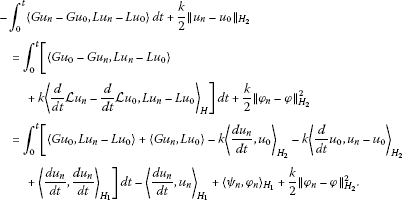From (2.1) and (3.12), we haveThen we get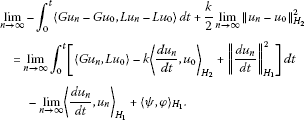(3.14)

In view of (3.9), (3.12), we obtain for all $v\in {\bigcup }_{n=1}^{\mathrm{\infty }}{\stackrel{˜}{X}}_{n}$

$\begin{array}{rcl}\underset{n\to \mathrm{\infty }}{lim}{\int }_{0}^{t}〈G{u}_{n},Lv〉\phantom{\rule{0.2em}{0ex}}dt& =& {\int }_{0}^{t}\left[k{〈\frac{d{u}_{0}}{dt},v〉}_{{H}_{2}}-{〈\frac{d{u}_{0}}{dt},\frac{dv}{dt}〉}_{{H}_{1}}\right]\phantom{\rule{0.2em}{0ex}}dt\\ +{〈\frac{d{u}_{0}}{dt},v〉}_{{H}_{1}}-{〈\psi ,v\left(0\right)〉}_{{H}_{1}}.\end{array}$
(3.15)

Since ${\bigcup }_{n=1}^{\mathrm{\infty }}{\stackrel{˜}{X}}_{n}$ is dense in ${W}^{1,2}\left(\left(0,T\right),{H}_{2}\right)\cap {L}^{p}\left(\left(0,T\right),{X}_{2}\right)$, $\mathrm{\forall }p<\mathrm{\infty }$, (3.15) holds for all $v\in {W}^{1,2}\left(\left(0,T\right),{H}_{2}\right)\cap {L}^{p}\left(\left(0,T\right),{X}_{2}\right)$. Thus, we have

$\begin{array}{rcl}\underset{n\to \mathrm{\infty }}{lim}{\int }_{0}^{t}〈G{u}_{n},L{u}_{0}〉\phantom{\rule{0.2em}{0ex}}dt& =& {\int }_{0}^{t}\left[k{〈\frac{d{u}_{0}}{dt},{u}_{0}〉}_{{H}_{2}}-{\parallel \frac{d{u}_{0}}{dt}\parallel }_{{H}_{1}}^{2}\right]\phantom{\rule{0.2em}{0ex}}dt\\ +{〈\frac{d{u}_{0}}{dt},{u}_{0}〉}_{{H}_{1}}-{〈\psi ,\phi 〉}_{{H}_{1}}.\end{array}$
(3.16)

From (3.12) and ${H}_{2}\subset {H}_{1}$ is compact imbedding, it follows that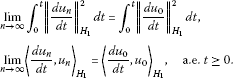Clearly,

$\underset{n\to \mathrm{\infty }}{lim}{\int }_{0}^{t}{〈\frac{d{u}_{n}}{dt},{u}_{n}〉}_{{H}_{1}}\phantom{\rule{0.2em}{0ex}}dt={\int }_{0}^{t}{〈\frac{d{u}_{0}}{dt},{u}_{0}〉}_{{H}_{1}}\phantom{\rule{0.2em}{0ex}}dt.$

Then (3.13) follows from (3.14)-(3.16), which imply assertion (1).

Secondly, we consider $G=A+B$. Let $v=\frac{d}{dt}L{u}_{n}$ in (3.9). In view of (2.2) and (3.1), it follows that

$\frac{1}{2}{\parallel \frac{d{u}_{n}}{dt}\parallel }_{{H}_{1}}^{2}+k{\int }_{0}^{t}{\parallel \frac{d{u}_{n}}{dt}\parallel }_{{H}_{2}}^{2}\phantom{\rule{0.2em}{0ex}}dt+F\left({u}_{n}\right)={\int }_{0}^{t}〈B\left({u}_{n}\right),\frac{d}{dt}L{u}_{n}〉\phantom{\rule{0.2em}{0ex}}dt+F\left({\phi }_{n}\right)+\frac{1}{2}{\parallel {\psi }_{n}\parallel }_{{H}_{1}}^{2}.$

From (3.3), we have

$\frac{1}{2}{\parallel \frac{d{u}_{n}}{dt}\parallel }_{{H}_{1}}^{2}+F\left({u}_{n}\right)+k{\int }_{0}^{t}{\parallel \frac{d{u}_{n}}{dt}\parallel }_{{H}_{2}}^{2}\phantom{\rule{0.2em}{0ex}}dt\le C{\int }_{0}^{t}\left[F\left({u}_{n}\right)+\frac{1}{2}{\parallel \frac{d{u}_{n}}{dt}\parallel }_{{H}_{1}}^{2}\right]\phantom{\rule{0.2em}{0ex}}dt+f\left(t\right),$
(3.17)

where $f\left(t\right)={\int }_{0}^{t}g\left(\tau \right)\phantom{\rule{0.2em}{0ex}}d\tau +\frac{1}{2}{\parallel \psi \parallel }_{{H}_{1}}^{2}+{sup}_{n}F\left({\phi }_{n}\right)$.

By using the Gronwall inequality, it follows that

$\frac{1}{2}{\parallel \frac{d{u}_{n}}{dt}\parallel }_{{H}_{1}}^{2}+F\left({u}_{n}\right)\le f\left(0\right){e}^{Ct}+{\int }_{0}^{t}f\left(\tau \right){e}^{C\left(t-\tau \right)}\phantom{\rule{0.2em}{0ex}}d\tau ,$
(3.18)

which implies that for all $0,

$\left\{{u}_{n}\right\}\subset {W}^{1,\mathrm{\infty }}\left(\left(0,T\right),{H}_{1}\right)\cap {L}^{\mathrm{\infty }}\left(\left(0,T\right),{X}_{2}\right)\phantom{\rule{1em}{0ex}}\text{is bounded}.$

From (3.17) and (3.18), it follows that

$\left\{{u}_{n}\right\}\subset {W}^{1,2}\left(\left(0,T\right),{H}_{2}\right)\phantom{\rule{1em}{0ex}}\text{is bounded}.$

Let

(3.19)

which implies that ${u}_{n}\to {u}_{0}$ in ${W}^{1,2}\left(\left(0,T\right),H\right)$ is uniformly weakly convergent from that ${H}_{2}\subset H$ is an compact imbedding.

The left proof is same as assertion (1).

Lastly, assume (3.6) holds. Let $v=\frac{{d}^{2}{u}_{n}}{{d}^{2}t}$ in (3.9). It follows that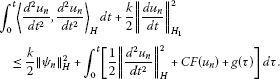From (3.18), the above inequality implies

(3.20)

We see that for all $0, $\left\{{u}_{n}\right\}\subset {W}^{2,2}\left(\left(0,T\right),H\right)$ is bounded. Thus, $u\in {W}^{2,2}\left(\left(0,T\right),H\right)$. □

## 4 Main result

We consider the strongly damped quasilinear wave equations (1.1). We give the following assumption for (1.1). There exists an ${C}^{1}$ function $F\left(x,\zeta \right)$, where $\zeta =\left\{{\zeta }_{\alpha }||\alpha |\le m\right\}$, ${\zeta }_{\alpha }$ corresponds to ${D}^{\alpha }u$ such that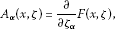(4.1)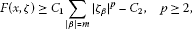(4.2)(4.3)

where $\lambda >0$, $\eta =\left\{{\eta }_{\beta }||\beta |=m\right\}$, $\xi =\left\{{\xi }_{\alpha }||\alpha |\le m-1\right\}$,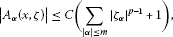(4.4)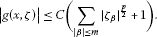(4.5)

Definition 4.1 We say $u\in {W}_{\mathrm{loc}}^{1,2}\left(\left(0,\mathrm{\infty }\right),{L}^{2}\left(\mathrm{\Omega }\right)\right)\cap {L}_{\mathrm{loc}}^{\mathrm{\infty }}\left(\left(0,\mathrm{\infty }\right),{W}_{0}^{m,p}\left(\mathrm{\Omega }\right)\right)$ is the weak solution of (1.1), if $u\left(0\right)=\phi$, and for $\mathrm{\forall }v\in {C}_{0}^{\mathrm{\infty }}\left(\mathrm{\Omega }\right)$, the following equality holds: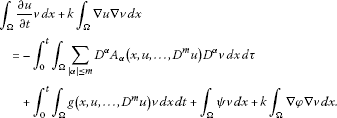(4.6)

Theorem 4.2 Under conditions (4.1)-(4.5), for$\left(\phi ,\psi \right)\in {W}_{0}^{m,p}\left(\mathrm{\Omega }\right)×{L}^{p}\left(\mathrm{\Omega }\right)$, there exists a global weak solution for (1.1)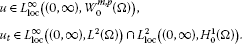Proof We introduce spatial sequences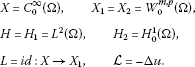Define map $G=A+B:{X}_{2}\to {X}_{1}^{\ast }$ by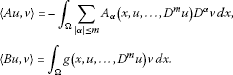We show that $G=A+B:{X}_{2}\to {X}_{1}^{\ast }$ is T-coercively weakly continuous. Let $\left\{{u}_{n}\right\}\subset {L}^{\mathrm{\infty }}\left(0,T,{W}^{2,p}\left(\mathrm{\Omega }\right)\cap {W}_{0}^{1,p}\left(\mathrm{\Omega }\right)\right)$ satisfying (2.7) and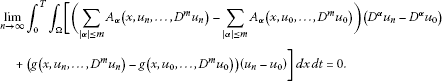(4.7)

We need prove that(4.8)

From (2.7) and Lemma 2.4, we obtain

(4.9)

We have the deformation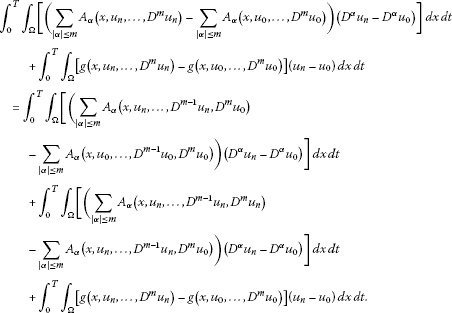(4.10)

From (4.9), (4.4), (4.5), and Lemma 2.5, we have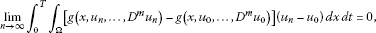(4.11)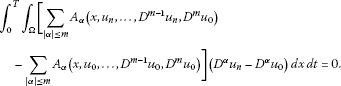(4.12)

From (4.7), (4.3), (4.10)-(4.12), it follows that

$\begin{array}{rcl}0& =& {\int }_{0}^{T}{\int }_{\mathrm{\Omega }}\left[\left(\sum _{|\alpha |\le m}{A}_{\alpha }\left(x,{u}_{n},\dots ,{D}^{m-1}{u}_{n},{D}^{m}{u}_{n}\right)\\ -\sum _{|\alpha |\le m}{A}_{\alpha }\left(x,{u}_{n},\dots ,{D}^{m-1}{u}_{n},{D}^{m}{u}_{0}\right)\right)\left({D}^{\alpha }{u}_{n}-{D}^{\alpha }{u}_{0}\right)\right]\phantom{\rule{0.2em}{0ex}}dx\phantom{\rule{0.2em}{0ex}}dt\\ \ge & \lambda {\int }_{0}^{T}{\int }_{\mathrm{\Omega }}{|{D}^{m}{u}_{n}-{D}^{m}{u}_{0}|}^{2}\phantom{\rule{0.2em}{0ex}}dx\phantom{\rule{0.2em}{0ex}}dt.\end{array}$

Since $\lambda >0$, we have

$\underset{n\to \mathrm{\infty }}{lim}{\int }_{0}^{T}{\int }_{\mathrm{\Omega }}{|{D}^{m}{u}_{n}-{D}^{m}{u}_{0}|}^{2}\phantom{\rule{0.2em}{0ex}}dx\phantom{\rule{0.2em}{0ex}}dt=0.$
(4.13)

From (4.9), (4.13), (4.4), (4.5), and Lemma 2.5, we get (4.8). Hence, $G=A+B:{X}_{2}\to {X}_{1}^{\ast }$ is T-coercively weakly continuous.

From (4.1) and (4.2), we get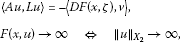which imply conditions (A1), (A2) of Theorem 3.1.

We will show (3.3) as follows. It follows that

$\begin{array}{rcl}|〈Bu,Lv〉|& =& {\int }_{\mathrm{\Omega }}|g\left(x,u,\dots ,{D}^{m}u\right)||v|\phantom{\rule{0.2em}{0ex}}dx\\ \le & \frac{k}{2}{\int }_{\mathrm{\Omega }}{|v|}^{2}\phantom{\rule{0.2em}{0ex}}dx+\frac{2}{k}{\int }_{\mathrm{\Omega }}{|g\left(x,u,\dots ,{D}^{m}u\right)|}^{2}\phantom{\rule{0.2em}{0ex}}dx\\ \le & \frac{k}{2}{\parallel v\parallel }_{{H}_{2}}^{2}+C{\int }_{\mathrm{\Omega }}{\left[\sum _{|\alpha |\le m}{|\zeta |}^{\frac{p}{2}}+1\right]}^{2}\phantom{\rule{0.2em}{0ex}}dx\\ \le & \frac{k}{2}{\parallel v\parallel }_{{H}_{2}}^{2}+CF\left(u\right)+C,\end{array}$

which implies condition (A3) of Lemma 2.4. From Lemma 2.4, Eq. (1.1) has a solution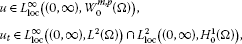satisfying□

## References

1. Webb GF: Existence and asymptotic behavior for a strongly damped nonlinear wave equation. Can. J. Math. 1980, 32: 631–643. 10.4153/CJM-1980-049-5

2. Carvalho AN, Cholewa JW: Local well posedness for strongly damped wave equations with critical nonlinearities. Bull. Aust. Math. Soc. 2002, 66: 443–463. 10.1017/S0004972700040296

3. Carvalho AN, Cholewa JW: Attractors for strongly damped wave equations with critical nonlinearities. Pac. J. Math. 2002, 207: 287–310. 10.2140/pjm.2002.207.287

4. Cholewa JW, Dlotko T: Strongly damped wave equation in uniform spaces. Nonlinear Anal. 2006, 64: 174–187. 10.1016/j.na.2005.06.021

5. Ghidaglia JM, Marzocchi A: Longtime behaviour of strongly damped wave equations, global attractors and their dimensions. SIAM J. Math. Anal. 1991, 22: 879–895. 10.1137/0522057

6. Massatt P: Longtime behaviour of strongly damped nonlinear wave equations. J. Differ. Equ. 1983, 48: 334–349. 10.1016/0022-0396(83)90098-0

7. Temam R: Infinite Dimensional Dynamical Systems in Mechanics and Physics. 2nd edition. Springer, New York; 1997.

8. Zhou S: Global attractor for strongly damped nonlinear wave equations. Funct. Differ. Equ. 1999, 6: 451–470.

9. Zhou S, Fan X: Kernel sections for non-autonomous strongly damped wave equations. J. Math. Anal. Appl. 2002, 275: 850–869. 10.1016/S0022-247X(02)00437-7

10. Fan X, Zhou S: The inflated attractors of non-autonomous strongly damped wave equations. Acta Math. Appl. Sinica (Engl. Ser.) 2004, 20(4):547–556. 10.1007/s10255-004-0192-9

11. Li H, Zhou S: Global periodic attractor for strongly damped and driven wave equations. Acta Math. Appl. Sinica (Engl. Ser.) 2006, 22(1):75–80. 10.1007/s10255-005-0287-y

12. Belleri V, Pata V:Attractors for semilinear strongly damped wave equation on ${R}^{3}$. Discrete Contin. Dyn. Syst. 2001, 7: 719–735.

13. Carvalho AN, Cholewa JW: Attractors for strongly damped wave equations with critical nonlinearities. Pac. J. Math. 2002, 207: 287–310. 10.2140/pjm.2002.207.287

14. Ghidaglia JM, Marzocchi A: Longtime behaviour of strongly damped wave equations, global attractors and their dimension. SIAM J. Math. Anal. 1991, 22: 879–895. 10.1137/0522057

15. Massat P: Limiting behavior for strongly damped nonlinear wave equations. J. Differ. Equ. 1983, 48: 334–349. 10.1016/0022-0396(83)90098-0

16. Yang H, Liu F: Boundary value problem for quasilinear wave equation. J. Math. Study 1999, 32(2):156–160.

17. Greenberg JM, Li T: The effect of boundary value problem for the quasilinear wave equation. J. Differ. Equ. 1984, 52: 66–75. 10.1016/0022-0396(84)90135-9

18. Li T Research Appl. Math. 32. In Global Classical Solutions for Quasilinear Hyperbolic Systems. Masson/Wiley, New York; 1994.

19. Li T, Qin T: Global smooth solutions for a class of quasilinear hyperbolic systems with dissipation. Chin. Ann. Math., Ser. B 1985, 6: 199–210.

20. Li T, Yu W Duke University Mathematics Series 5. In BVPs for Quasilinear Hyperbolic Systems. Duke University Press, Durham; 1985.

21. Alinhac S: The null condition for quasilinear wave equations in two space dimensions I. Invent. Math. 2001, 145: 597–618. 10.1007/s002220100165

22. Alinhac S: The null condition for quasilinear wave equations in two space dimensions II. Am. J. Math. 2001, 123(6):1071–1101. 10.1353/ajm.2001.0037

23. Zhang W: Cauchy problem for quasilinear hyperbolic systems with higher order dissipative terms. Acta Math. Appl. Sinica (Engl. Ser.) 2003, 19(1):71–82. 10.1007/s10255-003-0082-6

24. Metcalfe J, Sogge CD: Hyperbolic trapped rays and global existence of quasilinear wave equations. Invent. Math. 2005, 159: 75–117. 10.1007/s00222-004-0383-2

25. Yin H: The asymptotic behaviour of solutions for a class of quasilinear wave equations with cubic nonlinearity in two space dimensions. Acta Math. Appl. Sin. 2000, 16(3):299–312. 10.1007/BF02679895

26. Weidemaier P: Existence of regular solutions for a quasilinear wave equation with the third boundary condition. Math. Z. 1985, 191: 449–465.

27. Chen G, Yang Z, Zhao Z: Initial value problems and first boundary problems for a class of quasilinear wave equations. Acta Math. Appl. Sin. 1993, 9(4):289–301. 10.1007/BF02005918

28. Grille A: On the numerical solution of quasilinear wave equation with strong dissipative term. Appl. Math. Mech. 2004, 25(7):806–811. 10.1007/BF02437573

29. Chen F, Guo B, Wang P: Long time behavior of strongly damped nonlinear wave equations. J. Differ. Equ. 1998, 147: 231–241. 10.1006/jdeq.1998.3447

30. Ma T: Theories and Methods for Partial Differential Equations. Science Press, Beijing; 2011. Chinese

## Acknowledgements

The authors are very grateful to the anonymous referees whose careful reading of the manuscript and valuable comments enhanced the presentation of the manuscript. Foundation item: the National Natural Science Foundation of China (No. 11071177), the NSF of Sichuan Science and Technology Department of China (No. 2010JY0057), and the NSF of the Sichuan Education Department of China (No. 11ZA102).

## Author information

Authors

### Corresponding author

Correspondence to Hong Luo.

### Competing interests

The authors declare that they have no competing interests.

### Authors’ contributions

All authors typed, read and approved the final manuscript.

## Rights and permissions

Reprints and Permissions

Luo, H., Li, Lm. & Ma, T. Existence of solutions to strongly damped quasilinear wave equations. Adv Differ Equ 2012, 139 (2012). https://doi.org/10.1186/1687-1847-2012-139

• Accepted:

• Published:

• DOI: https://doi.org/10.1186/1687-1847-2012-139

### Keywords

• existence
• solution
• wave equations
• strongly damped
• quasi-linear